Journal of Measurements in Engineering

Published: 31 March 2019

# Reliability of vibration based tests for masonry compactness evaluation in sensitive case studies

Salvatore Russo1
1Iuav University of Venice, Venice, Italy
Editor's Pick
Views 121

#### Abstract

The reliability evaluation of vibration based (VB) tests in compactness evaluation of masonry bearing parts in sensitive case study as monuments and historic construction, is here proposed. The scientific aim is through a comparison between a lot of experimental data in situ relieved for different construction with different building age and level of conservation. In detail the investigation focuses on sonic device employed in direct and indirect way, whose use is very strategic in structural conservation. Final diagrams outline sonic wave trend in function of century, loads applied in investigated masonry structural part and in combination with similar non-destructive (ND) devices as the rebound hammer.

## 1. Introduction

The non-destructive devices and techniques vibration based (VB) originally employed in the mechanical engineering field to evaluate the welded steel joints efficiency are by now widely adopted and useful in the field of structural restoration of historic constructions and monuments [1, 2]. That is with the aim of improving the level of knowledge of the construction without invasive action [3, 4]. The use of this kind of nondestructive analysis is also suggested to plan then the more proper interventions of reinforcement or rehabilitation. The vibration as means for investigation could be considered not only as induced one but also as unknown input as in the case of natural vibration as they could be the wind or the pedestrian traffic or ‘urban noises’ which are reordered and then elaborated as output. In detail, sonic and ultrasonic test as well as the micro seismic which can be considered the vibration based (VB) tests are often employed to understand in masonry structural bearing parts as columns, arches, vaults or structural joints, their effective state of conservation and/or decay through the categories of technical judge as the compactness and homogeneity level are. Besides, the VB nondestructive tests are used also to estimate the presence of any void or incoherence or heterogeneity in the masonry thickness without any invasive action . The acquired qualitative information result in term of judge’s quality and not as mechanical or stresses or strains parameter with specific dimension value; equally they are extremely important since that they could affect the potential expected strength of masonry and allow a more detailed knowledge of the structure and its material . Nevertheless, even if the use of nondestructive VB techniques to understand the compactness value of masonry bearing parts is by now consolidated, its reliability and level of spendable are still in discussion if compared with data obtained through in situ micro-destructive or destructive tests or carried out in proper labs. The not yet friendly use of the VB tests is due probably also to the too high presence of masonry’ typology especially in some European countries and to the mathematic support, specifically the Fourier Analysis approach which is not direct and need elaboration through with it is possible to transform the acquired vibration input in velocity and then in frequency. Another point in discussion is also related to the aleatory of the manually induced vibration in the case of instrumented hammer. By the way, the use of natural and/or human vibrations from another side remains still aleatory since that originally the vibration is unknown . Besides also the more proper choice of the sensors in the case of a dynamic monitoring action considered as an extension of the VB test typology could affect quality and reliability of the output. The final aim of the proposed research is to compare several results both in term of velocity and frequency to evaluate the parameter’s sensitivity and to propose a final potential relation between compactness, age of masonry and velocity.

## 2. Current literature and technical recommendations

The use of VB test for diagnosis in structures and construction is by now supported by founded technical document and specific recommendations [8, 9]. The employment of VB devices is in function of the type of material. Usually but not strictly the sonic test is useful for modern and very old brick-mortar masonry or masonry assembled with stone instead of brick or in presence of masonry intended as a heterogeneous material as a consequence of different layers and component. The use of ultrasonic test is preferable in presence of concrete as well as the micro seismic test. The more consolidated technical recommendations for the uses of the VB test are cited in [3, 7, 10]. A typical scheme of application of VB (sonic and ultrasonic test) is evidenced in Fig. 1 in the case of different position of sensors .

Fig. 1A, B and C details show sonic test with instrumented hammer and one probe; details D indicate ultrasonic test with two probes and a picture of masonry wall with a typical crack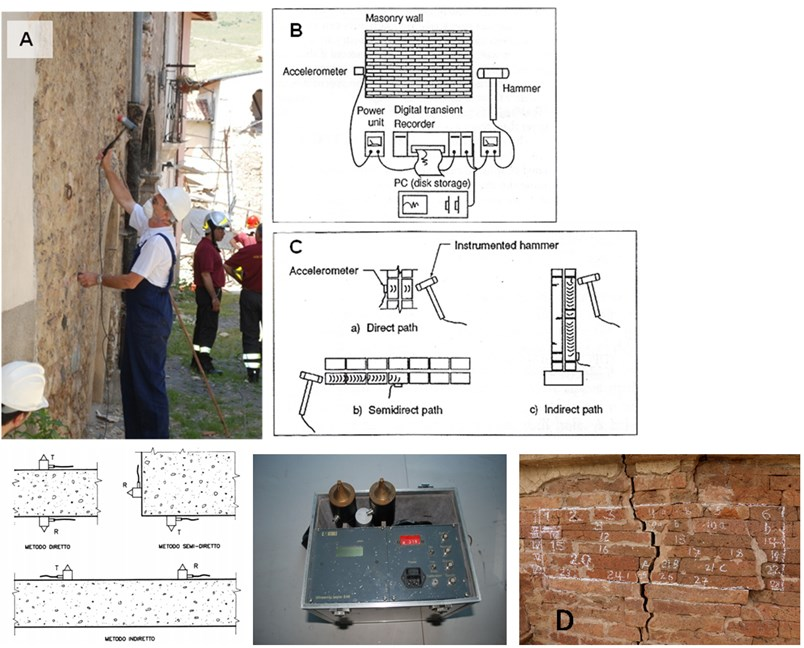On the top of Fig. 1, the details A, B and C show the use of VB sonic test with the instrumented hammer as known induced vibration with the general scheme indicated in B and the potential configuration available showed in C. On the bottom, detail D, is indicated the VB ultrasonic device with two probes and an example of damaged masonry wall [12, 13]. The more common and fruitful use of both VB methodology test is in presence of the instrumented hammer (or one probe) and the other probe (or sensor) in the opposite side than the thickness wall. With this configuration indeed is possible to understand the level of the compactness, and consequently of decay of the masonry through the velocity’s level of the sonic input. The flow chart showed in Fig. 2 indicates the velocity trend intended as a masonry compactness’s indicator. Currently the VB test are also used to detect damages and specifically the depth of cracks. In this case the measure through VB test will be related to the velocity of the sonic wave between two sensors located on the same plain but at opposite side than the investigated crack. In this general frame also, the dynamic monitoring action through sensor accelerometers could be considered as an extended application of the VB test. In that case both induced or natural available vibration could be used [5, 6].

Fig. 2Flow-chart related to the step-process elaboration (I-V) for velocity calculation with VB tests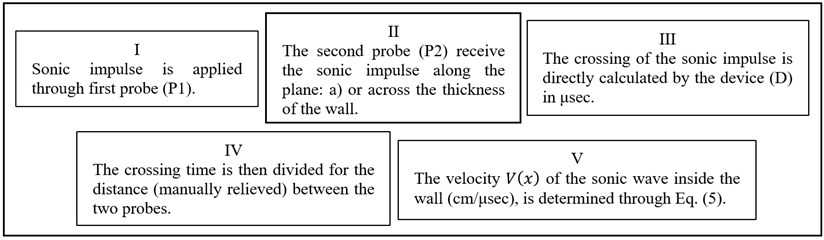## 3. Analytical approach and physical assumptions for VB test

The analytical approach for VB nondestructive test is mainly founded on the Fourier Transform Equations ; commonly its easier application is the Fast Fourier Transform one, commonly known through the acronym FFT. These equations allow to transform and elaborate the signal reordered by probe/sensor in sensitive data, as well as velocity, displacement and frequency . The relation between crossing velocity and masonry compactness level is such that higher velocities correspond to more compact masonry walls and, consequently, a positive level of structural conservation.

### 3.1. Induced vibration (known input)

In presence of sonic and ultrasonic test, every excitation induced by instrumented hammer can be represented with a function type indicated as following :

1
$p\left(t\right)={a}_{0}+{\sum }_{j=1}^{\infty }{a}_{j}\mathrm{c}\mathrm{o}\mathrm{s}\left(j{\omega }_{0}t\right)+{\sum }_{j=1}^{\infty }{b}_{j}\mathrm{s}\mathrm{i}\mathrm{n}\left(j{\omega }_{0}t\right),$

where ${\omega }_{0}$ is the frequency of the fundamental harmonic as the following:

2
$\omega =\frac{2\pi }{{T}_{0}}.$

In this case, ${T}_{0}$ is the period and $t$ is the time in which the function is defined. Considering now Eq. (1), a simpler approach is used:

3
$p\left(t\right)=\frac{1}{2\pi }{\int }_{-\mathrm{\infty }}^{+\mathrm{\infty }}P\left(\omega \right)\cdot {e}^{-i\mathrm{\omega }t}dt,$

where:

4
$P\left(\omega \right)={\int }_{-\infty }^{+\infty }p\left(t\right)\cdot {e}^{-i\omega t}dt.$

Then the obtained sonic wave crossing time will be transformed in velocity with the following very simple relation:

5
$V\left(x\right)=\frac{D\left(x\right)}{\mu \mathrm{sec}\left(x\right)}.$

Indeed $V\left(x\right)$ will be the crossing speed value of the pulse inside the wall between the two sensors, with $\mu \mathrm{s}\mathrm{e}\mathrm{c}\left(x\right)$ the crossing time and with $D\left(x\right)$ is the distance between the sensors.

### 3.2. Ambient/natural vibration (unknown input)

In the case of dynamic monitoring through ambient vibration like wind, wave effect, pedestrian traffic, loss of grave or noises the process analysis is typically output-type, since that it is based on the analysis of the unknown input. A more common extraction method based on the series of data that represents the only system response, is applied on the sole input of the ambient vibration. By the way, the ratio between the system response and the excitation is given by matrix $H$, expressed by the following relationship :

6
$\left[H\left(\omega \right)\right]={\sum }_{i=1}^{n}\frac{\left({\nu }_{i}\right)⟨{l}_{i}^{T}⟩}{j\omega -{\lambda }_{i}}+\frac{\left({\nu }_{i}^{*}\right)⟨{l}_{i}^{H}⟩}{j\omega -{\lambda }_{i}},$

with $n$ is the number of modes; superscripts $*$ and $H$ denote the complex conjugate and the transposed complex conjugate, respectively; is the vector of the modal shape; $⟨{l}_{i}^{T}⟩\in C$ is the vector of the factors of modal participation; and ${\lambda }_{i}$ are the poles of the system, in relation with the natural frequencies ($\omega$, i.e., eigenvalues) and damping values ($\zeta$), that will be by means of the following relationship:

7
${\lambda }_{i}{\lambda }_{i}^{*}=-{\zeta }_{i}{\omega }_{i}±j\sqrt{1-{\zeta }_{i}^{2}}{\omega }_{i},$

within the representation of the dynamic system by means of the $H\left(\omega \right)$ matrix, the response of the system itself to a dynamic stress is represented in the domain of frequencies by the well-known matrix ${S}_{yy}\in {C}^{m×m}$ which is the output spectrum:

8
$\left[{S}_{yy}\left(j\omega \right)\right]=\left[H\left(\omega \right)\right]\left[{S}_{uu}\left(\omega \right)\right]{\left[H\left(\omega \right)\right]}^{H},$

where ${S}_{uu}\in {C}^{k×j}$ is the stress matrix. Assuming now that the input spectrum is of an unknown entity, the central term of Eq. (8) is actually constant, so we will obtain:

9
$\left[{S}_{yy}\left(j\omega \right)\right]=\left[H\left(\omega \right)\right]\left[{S}_{uu}\right]{\left[H\left(\omega \right)\right]}^{H}.$

Consequently, in next Eq. (10), is defined because the input data include the only response of the system, which is the operational factor of reference:

10

where is a function of both the modal parameters of the system and the constant input spectrum. The values of damping $\zeta$ were estimated using the “half-power bandwidth” method by the following equation:

11
$\mathrm{\varsigma }=\frac{{f}_{2}-{f}_{1}}{{f}_{1}+{f}_{2}},$

where ${f}_{1}$ and ${f}_{2}$ are the frequencies before and after the frequency value at which there is a peak amplitude $P$ of the Fourier spectrum, with amplitudes of $P$/$\sqrt{2}$.

## 4. Cases study proposed

The investigation is mainly in comparing between several results obtained from different significant case studies of historic constructions or monumental structures through VB sonic test for masonry compactness evaluation.

Fig. 3Sensitive case studies. a) Rialto bridge (Venice 2015-2016), b) ducal palace (Venice, 20010-2016), c) Phya-sa-shwegu temple, Bagan (Myanmar, 2015), d) Collemaggio church and, e) S. Pietro Coppito church, f) Spanish castle, (L’Aquila, 2010)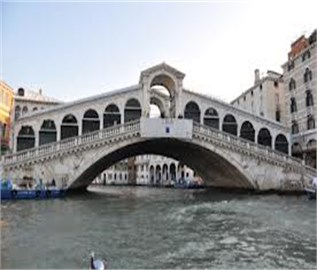a)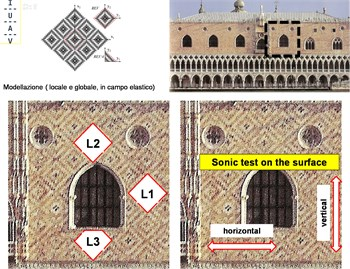b)c)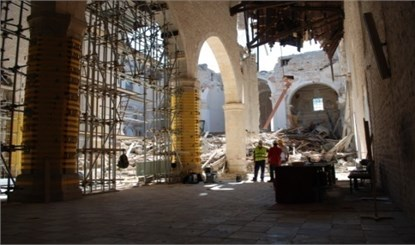d)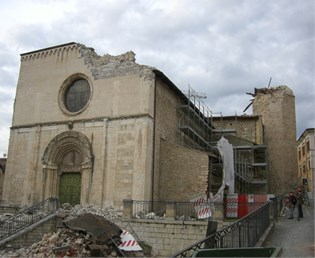e)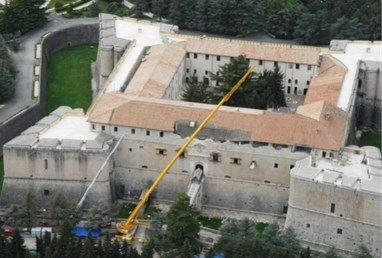f)

The proposed cases are related to the Rialto Bridge and Palazzo Ducale in Venice (Italy); to a Temple in Myanmar, exactly in the UNESCO area in Bagan, and to some cases of historic buildings and churches damaged by the 2009 earthquake occurred in L’Aquila (Italy). The common aspect is that all the investigated monument was made by masonry (brick-mortar type). A first summarizing of the proposed cases study is indicated in Fig. 3. For all buildings a specific campaign VB in situ tests has been carried out. Specifically, sonic test has been used to understand the level of effective structural decay of masonry bearing part through its compactness’s level.

## 5. Results and comparison

The results expressed in form of velocity and elaborated after formula application, from Eqs. (1)-(5), are now presented following for some sensitive case studies of Fig. 3. Outputs related to the central part of the Rialto Bridge deck have been presented in Figs. 4 and 5.

Fig. 4Design of the sonic test pattern at the key of the Rialto bridge in Venice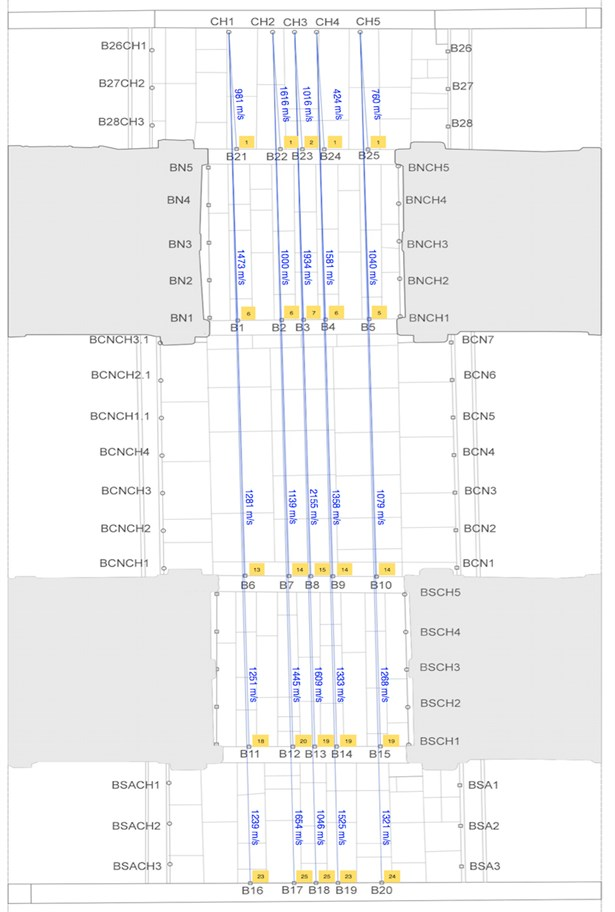Fig. 5Outputs in term of velocities in relation to five excitation points. (Ref. map Fig. 2)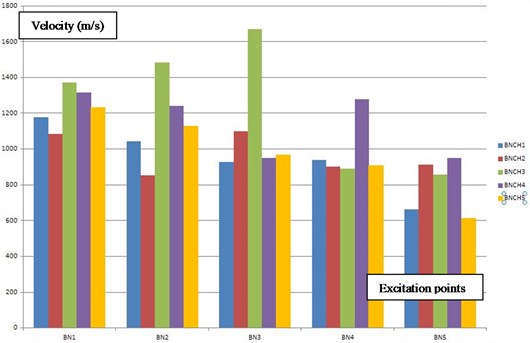In relation to Ducal Palace Façade, (see the general picture in detail b of Fig. 3), the more significant results obtained through the VB sonic test are presented in Fig. 6.

Fig. 6Results (velocity values) related to the Palazzo Ducale main facade in Venice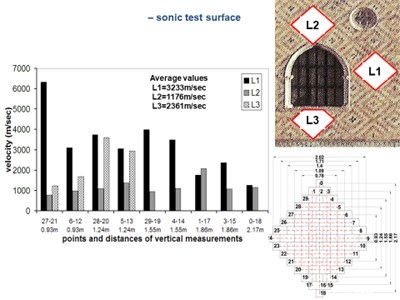a)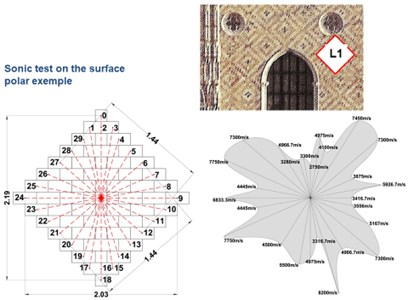b)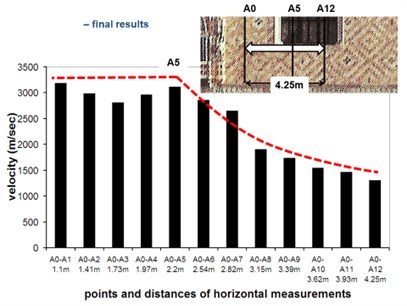c)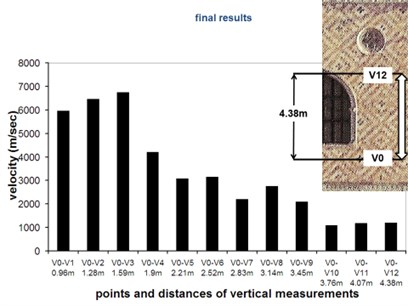d)

The relieved velocity reported in the $y$ axis in function of the position in the temples ($x$ axis) studied in Bagan (see detail c of Fig. 3) are indicated in Fig. 7. The symbol B means brick, while ‘H’ and ‘V’ indicate respectively the horizontal and vertical inspection through VB ultrasonic test across brick and mortar.

Fig. 7Results related to the temple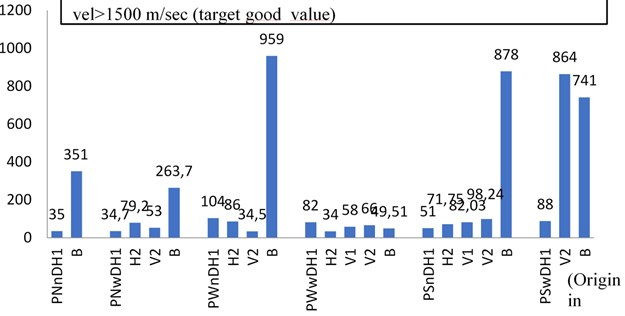Finally, for the historic construction in L’Aquila (Italy, after the 2009 Earthquake), the detailed in situ investigation carried out in the Collemaggio Basilica (see reference d) in Fig. 2), is reported in Fig. 8, while all the results related to the investigated buildings in L’aquila will be reported in next Chapter 6.

Fig. 8Results (velocity values) related to the pillars of the Collemaggio Basilica in L’aquila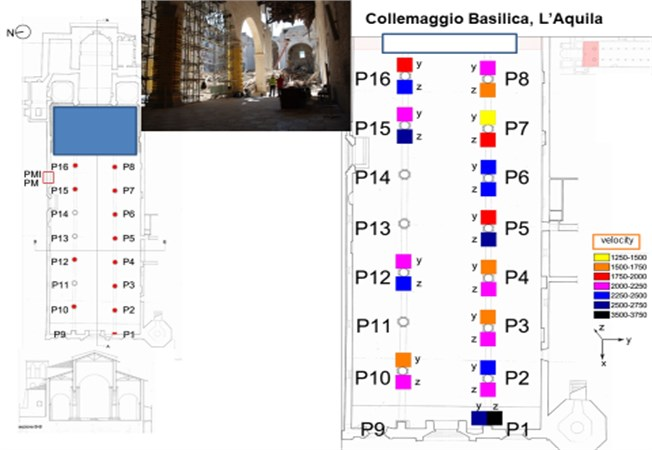## 6. Discussion on the reliability of VB test in presence of sensitive case studies

The results related to the investigation presented in the previous chapter evidenced many sensitive parameter and boundary conditions related to the too high variability of the velocity, the effect due to the damage and conservation level, the not negligible influence due to the load present during the test, and the age’s building influence in the investigated monument and historic construction. Due to the too high presence of parameters and variable, the discussion is now mainly focused on few points. By the way the tendency’s curves intended as correlation between velocity and compression value of bricks deduced by means rebound hammer, is showed in Fig. 9.

Bay the way Fig. 10 is dedicated to the variability of the velocity in function of the masonry bearing parts analyzed, while Fig. 11 shows finally the influence due to the age of the masonry in the variation of the velocity’s values.

Fig. 9Velocity’s distribution in relation to compression brick value in Bagan temple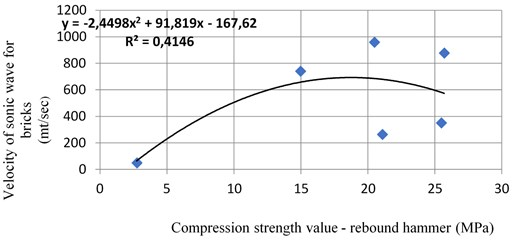a) Polynomial regression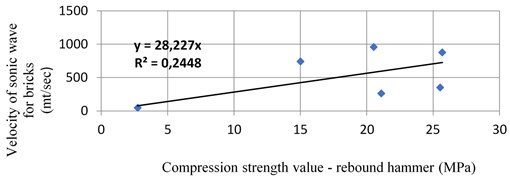b) Linear regression

Fig. 10Velocity’s distribution for masonry pillars, on the bottom, and walls, on the top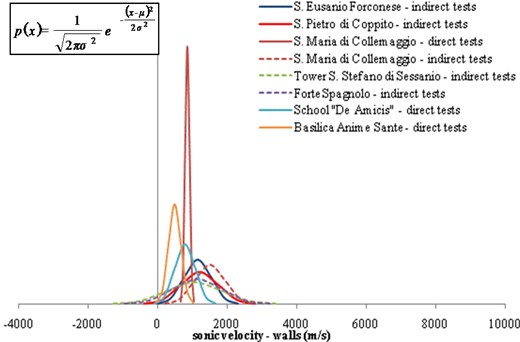a)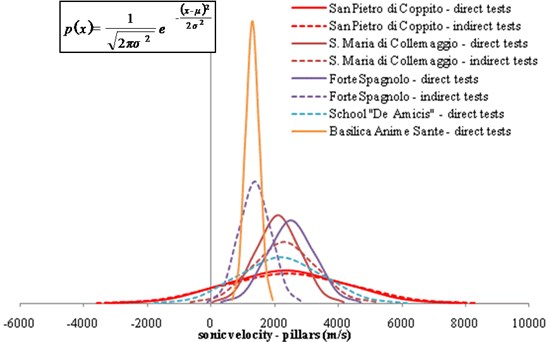b)

In detail Fig. 11 seems to outline a low tendency of decreasing of velocity with the decreasing of the age, and probably this point would have been expected more dominant.

Fig. 11Speed sonic wave vs the age of the historic constructions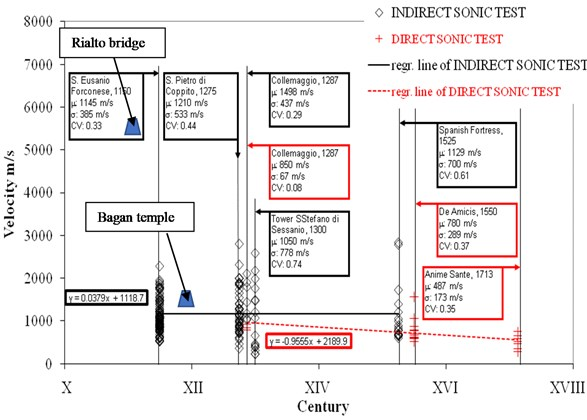## 7. Conclusions

On the basis of the proposed investigation, the following final considerations are proposed:

1) Sonic wave velocity evidenced, with few exceptions, a clear reduction tendency in presence of older masonry bearing parts.

2) A very interesting relation between sonic wave velocity and compressive strength value of masonry deduced with rebound hammer has been found as indicated in Fig. 9, with clear linear relation.

3) The load applied to the investigated masonry walls strongly influences the compactness’s values and velocity trend, with potential misunderstanding in term of overestimation of the expected strength of the masonry. By the way, the velocity distribution confirmed an evident influence due to the applied load and, more generally, higher value’s dispersion in presence of pillars than walls (see Fig. 10).

4) The relieved velocity values confirmed an influence due to the construction’s building age, as evidenced in Fig. 11, with a slight increase of the values for more recent constructions.

5) The analytical approach for VB test support confirmed its reliability also in the wider frame of the structural control health monitoring of sensitive case studies, as those here investigated.

#### Acknowledgements

The authors particularly thanks to technicians of the Lab of Strength of Materials of Iuav University of Venice in Venice (Italy), and Francesca Sciarretta for her support